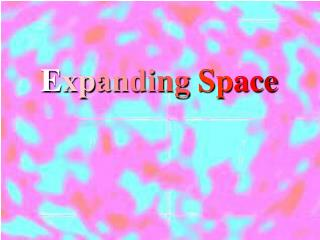DownloadDownload PresentationE xp an di ng Sp ac e

# E xp an di ng Sp ac e

Download Presentation## E xp an di ng Sp ac e

- - - - - - - - - - - - - - - - - - - - - - - - - - - E N D - - - - - - - - - - - - - - - - - - - - - - - - - - -
##### Presentation Transcript

1. ExpandingSpace

2. Olber’s Paradox: • In an infinite Universe… • Size • Age • Every line of sight would end on a star. • Every star would be observable. • The night sky would be very bright. • It would be pretty hot too…

3. Setting the stage: • General relativity predicts that the Universe must be either expanding or contracting. • Einstein thinks it should be static. • Introduces the cosmological constant to keep it static. • Friedmann determines that it still must be dynamic. • Einstein realizes that he is right, and calls the cosmological constant “The greatest mistake of my life”

4. Meanwhile: • Vesto Slipher at the Lowell Observatory in Flagstaff Arizona measures the red shift of spiral “Nebulae” • The redshift is the Doppler effect • 36 of 40 spiral nebulae are red shifted • Everything is moving away from “us”

5. Redshift - Doppler Effect If v << c: Δλ=v λ c Moving λ shorter in front λ longer in back (Kinda like a mullet) Sitting still λ same in all directions • Racecars going by • Bell on trains • Example • Java Applet

6. Put this in your notes: What is the change in wavelength of the 656 nm line of a galaxy receding at 20,000 km/s? What is the new wavelength? 20000E3/3E8*656 = 43.7333 nm 656 + 43.7333 = 699.733 nm 44 nm, 700 nm

7. What is the recession rate of a galaxy whose 656 nm line comes in at 691 nm? (approximate) (691-656)/656*3E5 = 16,000 km/s 16,000 km/s

8. Meanwhile: • Edwin Hubble uses the 100 inch telescope on Mt Wilson to look at “spiral nebulae”. • He can pick out individual stars • He finds Cepheids • He calculates the distance to and recession velocity of many galaxies. • There is a relationship

9. Hubble’s Law: v = Hd H = 71 km/s/Mpc Mpc = Mega parsecs The greater the distance, the greater the recession velocity.

10. An expanding universe: Before After

11. An expanding universe:

12. An expanding universe:

13. An expanding universe:

14. An expanding universe:

15. Put this in your notes: What is the distance to a galaxy that has a 480 nm line that comes in at 497 nm? (Use H = 71 km/s/Mpc ) (497-480)/480*3E5 = 10625 km/s 10625 km/s /(71 km/s/Mpc) = 149.6 Mpc 150 Mpc

16. What is the recession rate of a galaxy that is 26 Mpc away? (Use H = 71 km/s/Mpc ) 26 Mpc*(71 km/s/Mpc) = 1846 km/s 1846 km/s

17. What is the distance to the edge of the observable Universe? (Hint: What is the recession velocity if you can no longer see it?) (Use H = 71 km/s/Mpc ) 3E5km/s/(71 km/s/Mpc) = 4225 Mpc 4225 Mpc

18. What is the distance to a galaxy that has a 480 nm line that comes in at 497 nm? (Use H = 71 km/s/Mpc ) (497-480)/480*3E5 = 10625 km/s 10625 km/s /(71 km/s/Mpc) = 149.6 Mpc 150 Mpc